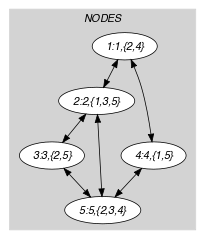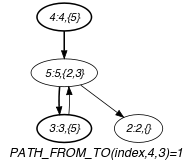## 5.316. path_from_to

Origin
Constraint

$\mathrm{𝚙𝚊𝚝𝚑}_\mathrm{𝚏𝚛𝚘𝚖}_\mathrm{𝚝𝚘}\left(\mathrm{𝙵𝚁𝙾𝙼},\mathrm{𝚃𝙾},\mathrm{𝙽𝙾𝙳𝙴𝚂}\right)$

Usual name

$\mathrm{𝚙𝚊𝚝𝚑}$

Arguments
 $\mathrm{𝙵𝚁𝙾𝙼}$ $\mathrm{𝚒𝚗𝚝}$ $\mathrm{𝚃𝙾}$ $\mathrm{𝚒𝚗𝚝}$ $\mathrm{𝙽𝙾𝙳𝙴𝚂}$ $\mathrm{𝚌𝚘𝚕𝚕𝚎𝚌𝚝𝚒𝚘𝚗}\left(\mathrm{𝚒𝚗𝚍𝚎𝚡}-\mathrm{𝚒𝚗𝚝},\mathrm{𝚜𝚞𝚌𝚌}-\mathrm{𝚜𝚟𝚊𝚛}\right)$
Restrictions
 $\mathrm{𝙵𝚁𝙾𝙼}\ge 1$ $\mathrm{𝙵𝚁𝙾𝙼}\le |\mathrm{𝙽𝙾𝙳𝙴𝚂}|$ $\mathrm{𝚃𝙾}\ge 1$ $\mathrm{𝚃𝙾}\le |\mathrm{𝙽𝙾𝙳𝙴𝚂}|$ $\mathrm{𝚛𝚎𝚚𝚞𝚒𝚛𝚎𝚍}$$\left(\mathrm{𝙽𝙾𝙳𝙴𝚂},\left[\mathrm{𝚒𝚗𝚍𝚎𝚡},\mathrm{𝚜𝚞𝚌𝚌}\right]\right)$ $\mathrm{𝙽𝙾𝙳𝙴𝚂}.\mathrm{𝚒𝚗𝚍𝚎𝚡}\ge 1$ $\mathrm{𝙽𝙾𝙳𝙴𝚂}.\mathrm{𝚒𝚗𝚍𝚎𝚡}\le |\mathrm{𝙽𝙾𝙳𝙴𝚂}|$ $\mathrm{𝚍𝚒𝚜𝚝𝚒𝚗𝚌𝚝}$$\left(\mathrm{𝙽𝙾𝙳𝙴𝚂},\mathrm{𝚒𝚗𝚍𝚎𝚡}\right)$ $\mathrm{𝙽𝙾𝙳𝙴𝚂}.\mathrm{𝚜𝚞𝚌𝚌}\ge 1$ $\mathrm{𝙽𝙾𝙳𝙴𝚂}.\mathrm{𝚜𝚞𝚌𝚌}\le |\mathrm{𝙽𝙾𝙳𝙴𝚂}|$
Purpose

Select some arcs of a digraph $G$ so that there is still a path between two given vertices of $G$.

Example
$\left(\begin{array}{c}4,3,〈\begin{array}{cc}\mathrm{𝚒𝚗𝚍𝚎𝚡}-1\hfill & \mathrm{𝚜𝚞𝚌𝚌}-\varnothing ,\hfill \\ \mathrm{𝚒𝚗𝚍𝚎𝚡}-2\hfill & \mathrm{𝚜𝚞𝚌𝚌}-\varnothing ,\hfill \\ \mathrm{𝚒𝚗𝚍𝚎𝚡}-3\hfill & \mathrm{𝚜𝚞𝚌𝚌}-\left\{5\right\},\hfill \\ \mathrm{𝚒𝚗𝚍𝚎𝚡}-4\hfill & \mathrm{𝚜𝚞𝚌𝚌}-\left\{5\right\},\hfill \\ \mathrm{𝚒𝚗𝚍𝚎𝚡}-5\hfill & \mathrm{𝚜𝚞𝚌𝚌}-\left\{2,3\right\}\hfill \end{array}〉\hfill \end{array}\right)$

The $\mathrm{𝚙𝚊𝚝𝚑}_\mathrm{𝚏𝚛𝚘𝚖}_\mathrm{𝚝𝚘}$ constraint holds since within the digraph $G$ corresponding to the item of the $\mathrm{𝙽𝙾𝙳𝙴𝚂}$ collection there is a path from vertex $\mathrm{𝙵𝚁𝙾𝙼}=4$ to vertex $\mathrm{𝚃𝙾}=3$: this path starts from vertex 4, enters vertex 5, and ends up in vertex 3.

Typical
 $\mathrm{𝙵𝚁𝙾𝙼}\ne \mathrm{𝚃𝙾}$ $|\mathrm{𝙽𝙾𝙳𝙴𝚂}|>2$
Symmetry

Items of $\mathrm{𝙽𝙾𝙳𝙴𝚂}$ are permutable.

Keywords
Arc input(s)

$\mathrm{𝙽𝙾𝙳𝙴𝚂}$

Arc generator
$\mathrm{𝐶𝐿𝐼𝑄𝑈𝐸}$$↦\mathrm{𝚌𝚘𝚕𝚕𝚎𝚌𝚝𝚒𝚘𝚗}\left(\mathrm{𝚗𝚘𝚍𝚎𝚜}\mathtt{1},\mathrm{𝚗𝚘𝚍𝚎𝚜}\mathtt{2}\right)$

Arc arity
Arc constraint(s)
$\mathrm{𝚒𝚗}_\mathrm{𝚜𝚎𝚝}$$\left(\mathrm{𝚗𝚘𝚍𝚎𝚜}\mathtt{2}.\mathrm{𝚒𝚗𝚍𝚎𝚡},\mathrm{𝚗𝚘𝚍𝚎𝚜}\mathtt{1}.\mathrm{𝚜𝚞𝚌𝚌}\right)$
Graph property(ies)
$\mathrm{𝐏𝐀𝐓𝐇}_\mathrm{𝐅𝐑𝐎𝐌}_\mathrm{𝐓𝐎}$$\left(\mathrm{𝚒𝚗𝚍𝚎𝚡},\mathrm{𝙵𝚁𝙾𝙼},\mathrm{𝚃𝙾}\right)=1$

Graph model

Within the context of the Example slot, part (A) of Figure 5.316.1 shows the initial graph from which we choose to start. It is derived from the set associated with each vertex. Each set describes the potential values of the $\mathrm{𝚜𝚞𝚌𝚌}$ attribute of a given vertex. Part (B) of Figure 5.316.1 gives the final graph associated with the Example slot. Since we use the $\mathrm{𝐏𝐀𝐓𝐇}_\mathrm{𝐅𝐑𝐎𝐌}_\mathrm{𝐓𝐎}$ graph property we show on the final graph the following information:

• The vertices that respectively correspond to the start and the end of the required path are stressed in bold.

• The arcs on the required path are also stressed in bold.

The $\mathrm{𝚙𝚊𝚝𝚑}_\mathrm{𝚏𝚛𝚘𝚖}_\mathrm{𝚝𝚘}$ constraint holds since there is a path from vertex 4 to vertex 3 (4 and 3 refer to the $\mathrm{𝚒𝚗𝚍𝚎𝚡}$ attribute of a vertex).

##### Figure 5.316.1. Initial and final graph of the $\mathrm{𝚙𝚊𝚝𝚑}_\mathrm{𝚏𝚛𝚘𝚖}_\mathrm{𝚝𝚘}$ set constraint(a) (b)
Signature

Since the maximum value returned by the graph property $\mathrm{𝐏𝐀𝐓𝐇}_\mathrm{𝐅𝐑𝐎𝐌}_\mathrm{𝐓𝐎}$ is equal to 1 we can rewrite $\mathrm{𝐏𝐀𝐓𝐇}_\mathrm{𝐅𝐑𝐎𝐌}_\mathrm{𝐓𝐎}$$\left(\mathrm{𝚒𝚗𝚍𝚎𝚡},\mathrm{𝙵𝚁𝙾𝙼},\mathrm{𝚃𝙾}\right)=1$ to $\mathrm{𝐏𝐀𝐓𝐇}_\mathrm{𝐅𝐑𝐎𝐌}_\mathrm{𝐓𝐎}$$\left(\mathrm{𝚒𝚗𝚍𝚎𝚡},\mathrm{𝙵𝚁𝙾𝙼},\mathrm{𝚃𝙾}\right)\ge 1$. Therefore we simplify $\underline{\overline{\mathrm{𝐏𝐀𝐓𝐇}_\mathrm{𝐅𝐑𝐎𝐌}_\mathrm{𝐓𝐎}}}$ to $\overline{\mathrm{𝐏𝐀𝐓𝐇}_\mathrm{𝐅𝐑𝐎𝐌}_\mathrm{𝐓𝐎}}$.